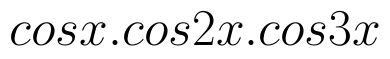Select Page

# CBSE 12 Science Answers for MCQ Maths Relations and Functions in English

CBSE 12 Science Answers for MCQ Maths Relations and Functions in English to enable students to get Answers in a narrative video format for the specific question.

Expert Teacher provides CBSE 12 Science Answers for MCQ Maths Relations and Functions through Video Answers in English language. This video solution will be useful for students to understand how to write an answer in exam in order to score more marks. This teacher uses a narrative style for a question from Relations and Functions not only to explain the proper method of answering question, but deriving right answer too.

Please find the question below and view the Answer in a narrative video format.

Question:

## Similar Questions from CBSE, 12th Science, Maths, Relations and Functions

Question 1 : Considergiven by f(x) = 4x + 3. Find the inverse of f. (View Answer Video)

Question 2 : The identity element for the binary operation * defined by a * b =, where a, b are the elements of a set of non-zero rational numbers, is, (View Answer Video)

Question 3 : Let A ={1, 2, 3}. Then number of equivalence relations containing (1, 2) is: (View Answer Video)

Question 4 : A functiondefined as f(x) = 5x is, (View Answer Video)

Question 5 : Let R be the relation in the set N given by :
R = {(a, b) : a = b - 2, b > 6}.

### Differential Equations

Question 1 : Find the general solution of differential equation(View Answer Video)

Question 2 : Find the solution of the differential equation(View Answer Video)

Question 3 : Write the differential equation representing the curvewhere a is an arbitrary constant. (View Answer Video)

Question 4 : Find the general solution of the following differential equation :(View Answer Video)

Question 5 : Solve the differential equation:(View Answer Video)

### Continuity and Differentiability

Question 1 : Differentiate the function w.r.t.x. (View Answer Video)

Question 2 : Differentiate the function w.r.t.x. (View Answer Video)

Question 3 : Findfor the function. (View Answer Video)

Question 4 : Ifand, find. (View Answer Video)

Question 5 : Differentiate the function w.r.t.x. (View Answer Video)

### Application of Integrals

Question 1 : Using integration, find the area of the region bounded by the triangle whose vertices are (-1, 2), (1, 5) and (3, 4). (View Answer Video)

Question 2 : Find the area of the smaller region bounded by the ellipseand the line(View Answer Video)

Question 3 : Find the area between the curves y = x and(View Answer Video)

Question 4 : Given that, for x = 0 to x = 20. Find f(x) and g(x) such that the area between f(x) andfrom x = 0 to x = 5 beand area between g(x) andfrom y = 0 to y = 5 be. Is? Like functions f and g which work is better, team work or individual work? (View Answer Video)

Question 5 : Using the method of integration, find the area of the region bounded by the lines: 5x - 2y -10 = 0, x + y - 9 = 0 and 2x - 5y - 4 = 0. (View Answer Video)